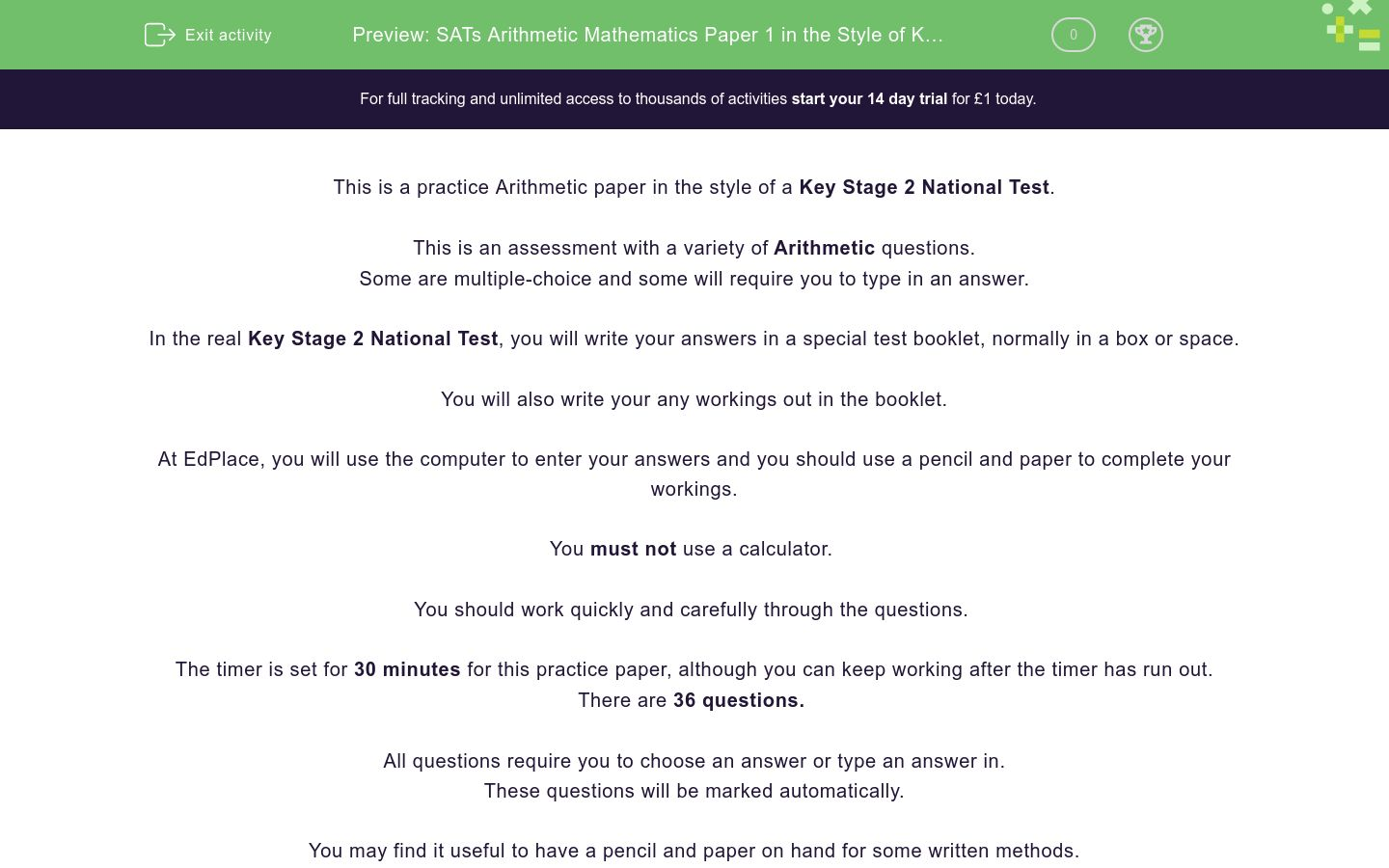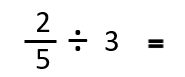# SATs Arithmetic Mathematics Paper 1 in the Style of Key Stage 2 National Tests (Practice 2)

In this assessment, students will be able to complete a timed Arithmetic paper in the style of Key Stage 2 SATs.Key stage:  KS 2

Curriculum topic:   SATs Practice Papers

Curriculum subtopic:   Arithmetic Practice Papers

Difficulty level:### QUESTION 1 of 10

This is a practice Arithmetic paper in the style of a Key Stage 2 National Test.

This is an assessment with a variety of Arithmetic questions.

Some are multiple-choice and some will require you to type in an answer.

In the real Key Stage 2 National Test, you will write your answers in a special test booklet, normally in a box or space.

You will also write your any workings out in the booklet.

At EdPlace, you will use the computer to enter your answers and you should use a pencil and paper to complete your workings.

You must not use a calculator.

You should work quickly and carefully through the questions.

The timer is set for 30 minutes for this practice paper, although you can keep working after the timer has run out.

There are 36 questions.

All questions require you to choose an answer or type an answer in.

These questions will be marked automatically.

You may find it useful to have a pencil and paper on hand for some written methods.

You may find some of the questions difficult.

If you are struggling to answer a question do not waste time on it, but move onto the next question.

Disclaimer:

We have no affiliation to the Standards and Testing Agency (STA) and these questions represent our own unique content developed by EdPlace Key Stage 2 Maths authors.

None of the content displayed here has been supplied by the STA or any other third party suppliers.

Calculate:

1469 - 457 = ?

Calculate:

1418 - 1015 = ?

Find the missing number.

? = 9348 + 48

FInd the missing number in the calculation below:

93 ÷ ? = 31

Find the missing number in the calculation below:

4 × ? = 360

Calculate:

60 × 30 × 10 = ?

Calculate:

13 × 12 × 10 = ?

Calculate:

69 × 4  = ?

Calculate:

93 × 8 = ?

Calculate:

438 × 7 = ?

Calculate:

5.72 + 27.5 = ?

Find the missing number in the calculation below:

24.3 - ? = 16.8

Calculate:

17.5 - 2.75 = ?

Calculate:

5786 ÷ 8 = ?

Show any remainders in the second box.

Calculate:

7321 ÷ 6 = ?

Show any remainders in the second box.

Calculate:

8.06 × 1000 = ?

Find the missing number in the calculation below:

13.4 × ? = 1340

Calculate:

243 - (14 - 10) = ?

Find the missing number in the calculation below:

(4 × 9) - ? = 28

Calculate:

10² ÷ 5² = ?

Calculate:

3³ + 2³ = ?

Calculate:

40% of 320.

Calculate:

80% of 350.

Find the missing fraction in this calculation:Find the missing numerator in the calculation:Find the missing fraction in this calculation:Find the missing fraction in this calculation:Calculate:Calculate:Calculate:Calculate:

1.3 × 9 = ?

Calculate:

2.4 × 5 = ?

Calculate:

370 × 27 = ?Calculate:

644 ÷ 28 = ?

Calculate:

405 ÷ 15 = ?

• Question 1

Calculate:

1469 - 457 = ?

EDDIE SAYS
The answer is 1012. 1469 is 1012 more than 1457. We can do this mentally by subtracting 457 from 469.
• Question 2

Calculate:

1418 - 1015 = ?

EDDIE SAYS
The answer is 403. We only really need to think about the difference between 18 and 15 here, which is 3.
• Question 3

Find the missing number.

? = 9348 + 48

EDDIE SAYS
The answer is 9396. We only really need to do 48 + 48 = 96 to solve this question.
• Question 4

FInd the missing number in the calculation below:

93 ÷ ? = 31

EDDIE SAYS
The missing number is 3. This is because 31 fits into 93 three times. You could count up in 31's to solve this.
• Question 5

Find the missing number in the calculation below:

4 × ? = 360

EDDIE SAYS
The missing number is 90. We know that 4 times 9 is 36 and that 360 is ten times larger than 36.
• Question 6

Calculate:

60 × 30 × 10 = ?

EDDIE SAYS
The missing number is 18000. Use the fact 6 × 3 = 18 to find that 60 × 30 = 1800. Multiply 1800 by ten, by moving each digit one place to the left.
• Question 7

Calculate:

13 × 12 × 10 = ?

EDDIE SAYS
This missing number is 1560. Use the multiplication fact 12 × 12 = 144, then add 12 to get 156. Make your answer ten times larger by moving each digit one place to the left.
• Question 8

Calculate:

69 × 4  = ?

EDDIE SAYS
The missing number is 276. This calculation can be done either by using short multiplication, or mentally as follows: First, multiply 70 by 4. This gives 280. Next, subtract 4 (because the question asks us to multiply 69 four times). This gives an answer of 276.
• Question 9

Calculate:

93 × 8 = ?

EDDIE SAYS
The missing number is 744. This calculation is best solved using short multiplication.
• Question 10

Calculate:

438 × 7 = ?

EDDIE SAYS
The missing number is 3066. This calculation is best solved using short multiplication.
• Question 11

Calculate:

5.72 + 27.5 = ?

EDDIE SAYS
The missing number is 33.22. Using column addition would be a reliable way to solve this calculation.
• Question 12

Find the missing number in the calculation below:

24.3 - ? = 16.8

EDDIE SAYS
The missing number is 7.5. Rearrange the numbers in the calculation, then find the difference between 24.3 and 16.8. 24.3 - 16.8 = 7.5
• Question 13

Calculate:

17.5 - 2.75 = ?

EDDIE SAYS
The answer is 14.75. When working with decimals of different lengths, we can use zero as a place holder to make things clearer. 17.50 - 2.75.
• Question 14

Calculate:

5786 ÷ 8 = ?

Show any remainders in the second box.

EDDIE SAYS
The answer is 723 r2. Use short division or 'bus stop' method to solve this one! There are 723 lots of 8 in 5786, with 2 remaining.
• Question 15

Calculate:

7321 ÷ 6 = ?

Show any remainders in the second box.

EDDIE SAYS
The answer is 1220 r1. This is because we can fit 1220 lots of 6 into 7321 with 1 remaining.
• Question 16

Calculate:

8.06 × 1000 = ?

EDDIE SAYS
The missing number is 8060. When multiplying by 1000, each digit in the number moves 3 spaces to the left. Don't forget that zero acts as a place holder!
• Question 17

Find the missing number in the calculation below:

13.4 × ? = 1340

EDDIE SAYS
The missing number is 100. When we multiply by 100, each digit moves two places to the left.
• Question 18

Calculate:

243 - (14 - 10) = ?

EDDIE SAYS
The answer is 239. First, solve the brackets. 14 - 10 = 4 Next, complete the calculation. 243 - 4 = 239
• Question 19

Find the missing number in the calculation below:

(4 × 9) - ? = 28

EDDIE SAYS
The missing number is 8. First, solve the brackets. 4 × 9 = 36 Next, complete the calculation by finding the difference between 36 and 38. 36 - 28 = 8
• Question 20

Calculate:

10² ÷ 5² = ?

EDDIE SAYS
The missing number is 4. First, find 10² which is 100. Next, find 5² which is 25. 100 ÷ 25 = 4.
• Question 21

Calculate:

3³ + 2³ = ?

EDDIE SAYS
The answer is 35. This is because 3 cubed is 27 (3 × 3 × 3) and 2 cubed is 8 (2 × 2 × 2). 27 + 8 = 35
• Question 22

Calculate:

40% of 320.

128
EDDIE SAYS
The answer is 128. We find 10% by dividing the number by 10. 10% of 320 is 32. 40% is 4 times larger than 10%. 32 × 4 = 128
• Question 23

Calculate:

80% of 350.

280
EDDIE SAYS
The answer is 280. To find 10%, divide the number by 10. 10% of 350 = 35 80% is 8 lots of 35. 8 × 35 = 280
• Question 24

Find the missing fraction in this calculation:EDDIE SAYS
The answer is 1/4. First, make both the fractions into 20ths. 13/20 - 8/20 = 5/20 5/20 can be simplified using the common factor of 4 to be shown as 1/4.
• Question 25

Find the missing numerator in the calculation:7
EDDIE SAYS
The missing numerator is 7. First, turn all the denominators into 10ths. 4 2/10 - 5/10 Next, turn the mixed number into an improper fraction. 42/10 - 5/10 = 37/10 Convert 37/10 into 3 7/10.
• Question 26

Find the missing fraction in this calculation:EDDIE SAYS
The answer is 2/15. When dividing fractions, we flip the second fraction and multiply. 3 whole ones can be shown as 3/1. So, 2/5 ÷ 3/1 becomes 2/5 ×1/3 = 2/15. The answer cannot be simplified.
• Question 27

Find the missing fraction in this calculation:EDDIE SAYS
The answer is 4/25. When multiplying fractions, we simply multiply the numerators and denominators and simplify if possible.
• Question 28

Calculate:EDDIE SAYS
The answer is 78. 1% of 600 is 6. 6 multiplied by 13 is 78.
• Question 29

Calculate:147
EDDIE SAYS
The answer is 147. First find 1/5. 245 ÷ 5 = 49 Next, find 3/5. 49 × 3 = 147
• Question 30

Calculate:310
EDDIE SAYS
The answer is 310. First, find one-seventh by dividing by 7 434 ÷ 7 = 62 Then, find five sevenths by multiplying by 5. 62 × 5 = 310
• Question 31

Calculate:

1.3 × 9 = ?

EDDIE SAYS
The answer is 11.7. An easy way to do this calculation mentally is: 1.3 × 10 = 13 13 - 1.3 = 11.7 You could also use short multiplication. Remember, if a calculation has 1 digit in the decimal place, then your answer must too.
• Question 32

Calculate:

2.4 × 5 = ?

EDDIE SAYS
The answer is 12. Any easy way to do this calculation is: 2.4 × 10 = 24 Half of 24 is 12. You could also do short multiplication, remembering that the calculation has one digit after the decimal place so the answer will too.
• Question 33

Calculate:

370 × 27 = ?

9990
EDDIE SAYS
The answer is 9990. Use long multiplication to solve this one.
• Question 345068
5,068
EDDIE SAYS
The answer is 5068. This question needs long multiplication. Take care with your written method first multiplying by 4, then by 10.
• Question 35

Calculate:

644 ÷ 28 = ?

23
EDDIE SAYS
The answer is 23. Use long division to solve this. It will help to write out multiples of 28 before beginning.
• Question 36

Calculate:

405 ÷ 15 = ?

27
EDDIE SAYS
The answer is 27. Use long division to solve this one, first writing out the multiples of 15.
---- OR ----

Sign up for a £1 trial so you can track and measure your child's progress on this activity.

### What is EdPlace?

We're your National Curriculum aligned online education content provider helping each child succeed in English, maths and science from year 1 to GCSE. With an EdPlace account you’ll be able to track and measure progress, helping each child achieve their best. We build confidence and attainment by personalising each child’s learning at a level that suits them.

Get started